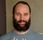cancel
Showing results for
Did you mean:Resolver I

## Filter not working as expected

Hi all. Iniatially posted this in the Dsktop section but wonder if it better fits here

I am trying to caculate the variance period on period per customer.

My Period value is in table date

My Customer value is in table customer

My Value is in table Sales Customer Period Value

customer   Period   Value

A                1            100

A                2             200

A                3             100

B                1              300

B                2              250

B                3              200

This Measure works as expected

Value1 = VAR periodvalue = value(SELECTEDVALUE('Date LookUp'[Financial Period])) Return Calculate('Sales Invoice History'[Gross Profit Post BDM], FILTER('Date LookUp','Date LookUp'[Financial Period] = periodvalue))

However This Measure returns blank

Value2 = VAR periodvalue = value(SELECTEDVALUE('Date LookUp'[Financial Period])) Return calculate(sum('Sales Invoice History'[STG_Gross_Profit_Less_BDM]), FILTER('Date LookUp','Date LookUp'[Financial Period] = periodvalue +1))

periodvalue + 1 is calulcating correctly and giving me the expected outcome so it's not that

If I take periodvalue + 1 and enter a hardcoded value I still get blank.

What have I done wrong here in my filter?

My plan was to do Value1 - Value2 to get the variance and drop the measure into my table to calculate by Customer

If anyone can help or shed some lighton where I am going wrong it would be appreciated.

Thanks.

1 ACCEPTED SOLUTIONSuper User

hi, @GarethWoodhouse ,

could you try to wrap an ALL() around 'Date Lookup' inside the filter, like this:

``````Value2 =
VAR periodvalue =
VALUE ( SELECTEDVALUE ( 'Date LookUp'[Financial Period] ) )
RETURN
CALCULATE (
SUM ( 'Sales Invoice History'[STG_Gross_Profit_Less_BDM] ),
FILTER (
ALL ( 'Date LookUp' ),
'Date LookUp'[Financial Period] = periodvalue + 1
)
)``````

Cheers,
Sturla

If this post helps, then please consider Accepting it as the solution. Kudos are nice too.

2 REPLIES 2Super User

hi, @GarethWoodhouse ,

could you try to wrap an ALL() around 'Date Lookup' inside the filter, like this:

``````Value2 =
VAR periodvalue =
VALUE ( SELECTEDVALUE ( 'Date LookUp'[Financial Period] ) )
RETURN
CALCULATE (
SUM ( 'Sales Invoice History'[STG_Gross_Profit_Less_BDM] ),
FILTER (
ALL ( 'Date LookUp' ),
'Date LookUp'[Financial Period] = periodvalue + 1
)
)``````

Cheers,
Sturla

If this post helps, then please consider Accepting it as the solution. Kudos are nice too.Resolver I

Initially this didn't work for me but I then realised it was because I also had a Year field and the All on the filter was bypassing this page filter. By adding a filter on selected year into the value2 code I got this working perfectly. Thanks all for your help. For anyone else experiencing similar problems here is the final code that works for my exmple

GP Variance =

VAR periodvalue1 = VALUE ( SELECTEDVALUE ( 'Date LookUp'[Financial Period] ) )

VAR Value1 = CALCULATE ( sum('Sales Invoice History'[STG_Gross_Profit_Less_BDM]), FILTER ( 'Date LookUp', 'Date LookUp'[Financial Period] = periodvalue1 ) )

VAR periodvalue2 = VALUE ( SELECTEDVALUE ( 'Date LookUp'[Financial Period] ) -1 )

VAR yearvalue2 = VALUE ( SELECTEDVALUE ( 'Date LookUp'[Financial Year] ) )

Var Value2 = CALCULATE ( sum('Sales Invoice History'[STG_Gross_Profit_Less_BDM]), FILTER ( all('Date LookUp'), 'Date LookUp'[Financial Period] = periodvalue2 && 'Date LookUp'[Financial Year] = yearvalue2 ) )

Return IF(Value2 <> 0,Value1 - Value2,0)Announcements#### Exclusive opportunity for Women!

Join us for a free, hands-on Microsoft workshop led by women trainers for women where you will learn how to build a Dashboard in a Day!#### Power Platform Conference-Power BI and Fabric Sessions

Join us Oct 1 - 6 in Las Vegas for the Microsoft Power Platform Conference.Top Solution Authors
Top Kudoed Authors
Users online (2,251)